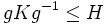# Order-dominating subgroup

This article defines a subgroup property: a property that can be evaluated to true/false given a group and a subgroup thereof, invariant under subgroup equivalence. View a complete list of subgroup properties[SHOW MORE]

## Definition

### Symbol-free definition

A finite subgroup of a group is termed order-dominating if every other subgroup whose order divides its order, is conjugate to a subgroup contained in it.

### Definition with symbols

Let$G$ be a group and$H$ be a finite subgroup. Then,$H$ is termed order-dominating in$G$ if, for any subgroup$K \le G$ such that the order of$K$ divides the order of$G$, there exists$g \in G$ such that$gKg^{-1} \le H$.